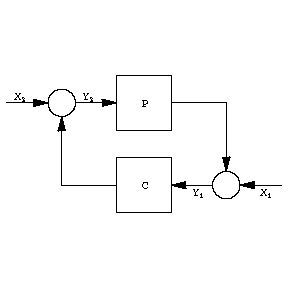# H^infinity-control-theory

A branch of control theory where the performance of a dynamical system (cf. Automatic control theory) is appraised in terms of the $H ^ \infty$- norm. The Banach space $H ^ \infty$( named after G.H. Hardy, cf. Hardy classes) consists of all complex-valued functions of a complex variable which are analytic and of bounded modulus in the open right half-plane. The norm of such a function is the supremum modulus:

$$\| F \| _ \infty = \sup _ { \mathop{\rm Re} s > 0 } | F( s) | .$$

By a theorem of Fatou (cf. Fatou theorem), such a function has a boundary value $F( i \omega )$ for almost-all $\omega$, and, moreover,

$$\| F \| _ \infty = \mathop{\rm esssup} _ \omega | F( i \omega ) | .$$

The theory of $H ^ \infty$ control was initiated by G. Zames [a1], [a2], [a3], who formulated a basic feedback problem as an optimization problem with an operator norm, in particular, an $H ^ \infty$- norm. Relevant contemporaneous works are those of J.W. Helton [a4] and A. Tannenbaum [a5].

The theory treats dynamical systems represented as integral operators of the form

$$y( t) = \int\limits _ { 0 } ^ { t } g( t- \tau ) x( \tau ) d \tau .$$

Here $g$ is sufficiently regular to make the input-output mapping $x \mapsto y$ a bounded operator on $L _ {2} [ 0 , \infty )$. Taking Laplace transforms gives $Y( s)= G( s) X( s)$. The function $G$ is called the transfer function of the system and it belongs to $H ^ \infty$ because the integral operator is bounded. Moreover, the $H ^ \infty$- norm of $G$ equals the norm of the integral operator, i.e.,

$$\tag{a1 } \| G \| _ \infty = \sup _ {\| x \| _ {2} \leq 1 } \ \| y \| _ {2} .$$

There are two prototype problems giving rise to an optimality criterion with the $H ^ \infty$- norm. The first is the problem of robust stability of the feedback systemFigure: h046020a

Here $P$ and $C$ are transfer functions in $H ^ \infty$, and $X _ {1}$, $X _ {2}$, $Y _ {1}$, $Y _ {2}$ are Laplace transforms of signals; $P$ represents a "plant" , the dynamical system which is to be controlled, and $C$ represents the "controller" (cf. also Automatic control theory). The figure stands for the two equations

$$Y _ {1} = X _ {1} + PY _ {2} ,\ Y _ {2} = X _ {2} + CY _ {1} ,$$

which can be solved to give

$$\left [ \begin{array}{c} Y _ {1} \\ Y _ {2} \end{array} \right ] = \ \left [ \begin{array}{cc} \frac{1 }{1- PC } & \frac{P }{1- PC } \\ \frac{C }{1- PC } & \frac{1 }{1- PC } \\ \end{array} \right ] \left [ \begin{array}{c} X _ {1} \\ X _ {2} \end{array} \right ] .$$

Therefore, the input-output mapping for the feedback system has four transfer functions. The feedback system is said to be internally stable if these four transfer functions are all in $H ^ \infty$. A simple sufficient condition for this is $\| PC \| _ \infty < 1$.

Internal stability is robust if it is preserved under perturbation of $P$. There are several possible notions of perturbation, typical of which is additive perturbation. So suppose $P$ is perturbed to $P+ \Delta P$, with $\Delta P$ in $H ^ \infty$. About $\Delta P$ it is assumed that only a bound on $| \Delta P( i \omega ) |$ is known, namely,

$$| \Delta P( i \omega ) | < | R( i \omega ) | ,\ \textrm{ a.a. } \ \omega ,$$

where $R \in H ^ \infty$. J.C. Doyle and G. Stein [a6] showed that internal stability is preserved under all such perturbations if and only if

$$\tag{a2 } \| RC( 1- PC) ^ {- 1 } \| _ \infty < 1 .$$

This leads to the robust stability design problem: Given $P$ and $R$, find $C$ so that the feedback system is internally stable and (a2) holds.

The second problem relates to the same feedback system. Suppose $X _ {2} = 0$, $X _ {1}$ represents a disturbance signal, and the objective is to reduce the effect of $X _ {1}$ on the output $Y _ {1}$. The transfer function from $X _ {1}$ to $Y _ {1}$ equals $( 1- PC) ^ {- 1 }$. Suppose, in addition, that the disturbance is not a fixed signal, but can be the output of another system with any input in $L _ {2} [ 0 , \infty )$ of unit norm; let this latter system have transfer function $W$ in $H ^ \infty$. Then, in view of (a1), the supremal $L _ {2} [ 0 , \infty )$- norm of $y _ {1}$ over all such disturbances equals $\| W( 1- PC) ^ {- 1 } \| _ \infty$. This leads to the disturbance attenuation problem: Given $P$ and $R$, find $C$ to achieve internal stability and minimize $\| W( 1- PC) ^ {- 1 } \| _ \infty$.

The above two problems are special cases of the more general standard $H ^ \infty$ control problem. It can be solved by reduction to the Nehari problem of approximating a function in $L _ \infty$( bounded functions on the imaginary axis) by one in $H ^ \infty$. A summary of this theory is in [a7], and a detailed treatment is in [a8].

How to Cite This Entry:
H^infinity-control-theory. Encyclopedia of Mathematics. URL: http://encyclopediaofmath.org/index.php?title=H%5Einfinity-control-theory&oldid=50664